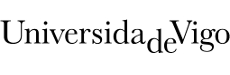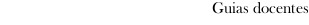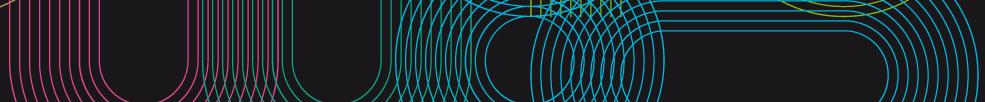Educational guide 2019_20Escola de Enxeñaría Aeronáutica e do Espazo
 Grao en Enxeñaría AeroespacialSubjectsFluid mechanicsContents
 Topic Sub-topic Introduction Subject 1. Introduction:Fundamental concepts of the Fluid mechanics. Solids, liquids and gases. The fluids like continuous means. Thermodynamic balance venue. Fluent particle. Speed, density and specific internal energy. *Viscosidad.Intensive fluent magnitudes and *extensivas. Equations of state. Balances of mass, quantity of movement and energy Subject 2. Cinematic of Fluids:Lagrangian and Eulerian descriptions. Lines, surfaces and fluent volumes. Paths and both. Lines of traces. Lines surfaces and tubes of current. Points of *remanso. Substantial derivative. Acceleration. Movements *estacionarios and uniform. Normal speed of advance of a surface. Flow *convectivo. *Derivación Of widespread integrals to fluent volumes. Theorem of the transport of *Reynolds. Open systems and enclosed systems. Relative movement in the surroundings of a point. Circulation. Irrotational movements. Theorem of *Bjerknes-Kelvin. Tensor of speeds of deformation. Subject 3. General equations:Principle of conservation of the mass. Equation of the in shape integral continuity. Equation of the in shape differential continuity. Function of current and material function. Equation of quantity of movement. Strengths of long scope. Strengths of surface or of short scope. Tensor of efforts. Equation of the quantity of movement in shape integral. Equation of the quantity of movement in shape differential. Law of *Navier-*Poisson. Tensor of viscous efforts. Equation of the in shape integral energy. Flow of heat by driving. Differential form of the equation of the energy. Law of Fourier. Flow of heat by driving. Summary of the equations of Fluid mechanics. Initial conditions. Conditions of outline more usual. Condition of no slide. *Fluidostática Subject 4. *Fluidostática:General equations. Conditions of balance. Potential function of mass strengths. Potential energy and principle of conservation of the energy. Probes of static pressure. Hydrostatics. Balance of gases. Standard atmosphere Dimensional analysis and Physical Similarity Subject 5. Dimensional analysis and Physical Similarity:Theorem *Pi of *Vaschy-*Buckingham. Solutions of similarity. Physical similarity. Adimensional numbers in Fluid mechanics Movements laminares and turbulent in tubes. Subject 6. Movement laminar unidirectional of fluent incompresibles: Current of Couette. Current of Poiseuille. Movement laminar in tubes. Losses of load in diet laminar. Factor of friction. Effect of the entrance. Subject 7. Movement to low numbers of Reynolds. Equations. Initial conditions and of outline. Application to fluent incompresibles. Movements around a cylinder and a sphere. Lubrication: Equation of Reynolds of the lubrication 3D. Applications. Cojinete Cylindrical, lubrication with gases, patín rectángular, others. Subject 8. Turbulent movement in tubes: Introduction to the turbulent movement of fluent incompresibles in tubes. Unsteadiness of the flow laminar in tubes. Losses of load in turbulent diet. Factor of friction. Diagram of Moody Introducción a fluidos ideales. Tema 9. Fluidos ideales. Ecuaciones de Euler: Introducción. Flujos a altos números de Reynolds. Ecuación de Bernoulli. Sondas Pitot. Condiciones de remanso. Movimiento casi estacionario. Prácticas de laboratorio Resolución de problemas de los temas expuestos en Aula. Visualización de flujos a bajos números de Reynolds. Visualización de calles de Karman tras distintos objetos romos. Observación de cambios de frecuencia de la estela. Separación de capa límite. Transición de flujo laminar a flujo turbulento. Ensayo en banco aerodinámico: Medición de velocidades en chorro de aire. Comprobación de la ecuación de Bernoulli Ensayo en túnel de viento: Distribución de presiones alrededor de un cilindro. Cálculo del coeficiente de resistencia. Distribución de presiones alrededor de un perfil de ala. Cálculo del coeficiente de sustentación. Medición tubo de Prandtl. Medición con tubo Pitot
 Universidade de Vigo            | Reitoría | Campus Universitario | C.P. 36.310 Vigo (Pontevedra) | Spain | Tlf: +34 986 812 000